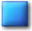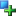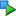##Image_Graph_Data_Line

Description

Line data-element

This class is used to draw a line in the diagram. Support for stacking is implemented in this element.

Located in /Graph/Data/Line.php (line 45)

```Image_Graph_Data_Common
|
--Image_Graph_Data_Line```
Method SummaryImage_Graph_Data_Line Image_Graph_Data_Line (object Image_Graph &\$parent, array \$data, [array \$attributes = array()])void drawGD (resource &\$img, [int \$drawWhat = IMAGE_GRAPH_DRAW_FILLANDBORDER])void stackingDrawGD (array &\$dataElements, mixed &\$img)void stackingPrepare (array &\$dataElements)
MethodsConstructor Image_Graph_Data_Line (line 55)

Constructor

• access: public
Image_Graph_Data_Line Image_Graph_Data_Line (object Image_Graph &\$parent, array \$data, [array \$attributes = array()])
• object Image_Graph &\$parent: parent object
• array \$data: numerical data to be drawn
• array \$attributes: attributes like colordrawGD (line 126)

Draws diagram element

The parameter \$drawWhat is needed to be able to first draw the fill of all line-elements and later draw the lines, in case that stacking is used.

• access: public
void drawGD (resource &\$img, [int \$drawWhat = IMAGE_GRAPH_DRAW_FILLANDBORDER])
• resource &\$img: GD-resource to draw to
• int \$drawWhat: choose what to draw; use constants IMAGE_GRAPH_DRAW_FILLANDBORDER, IMAGE_GRAPH_DRAW_JUSTFILL or IMAGE_GRAPH_DRAW_JUSTBORDER

Redefinition of:
Image_Graph_Data_Common::drawGD()
Draws diagram elementstackingDrawGD (line 105)

Draw all diagram elements in this stacking-group

The specific data-type-class knows best how to draw the data-elements of this type. If stacking is used this function will be called instead of drawGD(). The best results for drawing lines are achieved by first drawing the fill of each element and afterwards drawing the lines. This is the best solution for the resulting image.

• static:
• access: public
void stackingDrawGD (array &\$dataElements, mixed &\$img)
• array &\$dataElements: references to dataElements (objects of this type)

Redefinition of:
Image_Graph_Data_Common::stackingDrawGD()
Draw all diagram elements in this stacking-groupstackingPrepare (line 75)

Prepare given dataElements of this type for stacking

This function is called from inside the Image_Graph-baseinstance to stack elements of this data-type. This is done for every data-type in it's respective classes separately because stacking might depend on the type of data-representation. This method is called statically and receives a list of references to objects of this data-type. Using the references it can directly access all methods and attributes of each object.

• static:
• access: public
void stackingPrepare (array &\$dataElements)
• array &\$dataElements: references to dataElements (objects of this type)

#### Inherited Methods

Inherited From Image_Graph_Data_CommonImage_Graph_Data_Common::Image_Graph_Data_Common()Image_Graph_Data_Common::drawGD()Image_Graph_Data_Common::setColor()Image_Graph_Data_Common::setDataMarker()Image_Graph_Data_Common::setFill()Image_Graph_Data_Common::stackingDrawGD()

Documentation generated on Sat, 21 Feb 2004 23:28:06 +0100 by phpDocumentor 1.3.0RC2